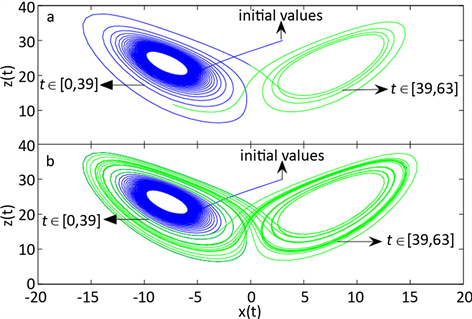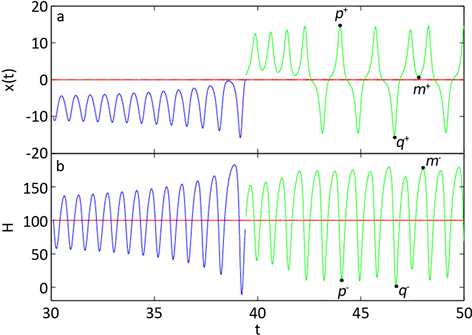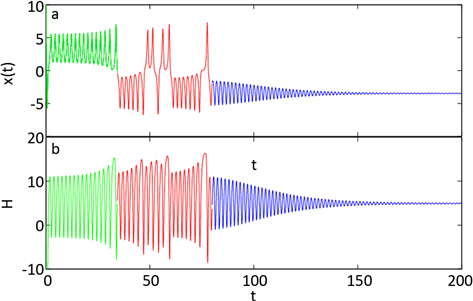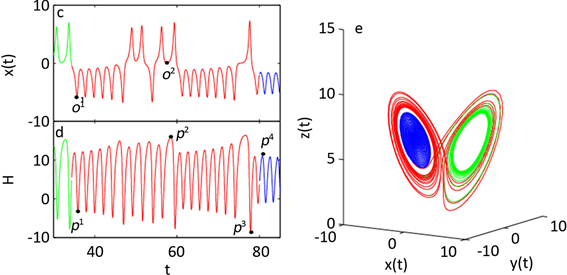# 具有隐藏吸引子的广义Lorenz系统动力学分析Dynamics Analysis of Generalized Lorenz System with Hidden Attractors

• 全文下载: PDF(1197KB)    PP.1979-1985   DOI: 10.12677/AAM.2019.812227
• 下载量: 141  浏览量: 206

In this paper, a generalized Lorenz system with hidden attractors is introduced. The dynamic phenomena including periodic motion, chaotic state and transient chaos is observed when different initial states are given. It is found that the dynamic behavior of the generalized Lorenz system depends on the system parameters and initial states. Through a series of calculations, the Hamiltonian energy function of the system is given, and the energy conversion in different motion states is analyzed.

1. 引言

2. 具有隐藏吸引子的广义Lorenz系统

$\left\{\begin{array}{l}\stackrel{˙}{x}=-a\left(x-y\right)-byz\\ \stackrel{˙}{y}=cx-y-xz\\ \stackrel{˙}{z}=-dz+xy\end{array}$ (1)

3. 具有隐藏吸引子的广义Lorenz系统的Hamilton能量

$\stackrel{˙}{x}=f\left(x\right)$ (2)

$f\left(x\right)={f}_{c}\left(x\right)+{f}_{d}\left(x\right)$ (3)

$\nabla {H}^{\text{T}}{f}_{c}\left(x\right)=0$ (4)

$\stackrel{˙}{H}=\frac{\text{d}H}{\text{d}t}=\nabla {H}^{\text{T}}{f}_{d}\left(x\right)$ (5)

${f}_{c}\left(x\right)=\left(\begin{array}{c}ay-byz\\ cx-xz\\ xy\end{array}\right)，\text{\hspace{0.17em}}{f}_{d}\left(x\right)=\left(\begin{array}{c}-ax\\ -y\\ -dz\end{array}\right)$ (6)

$\left(ay-byz\right)\frac{\partial H}{\partial x}+\left(cx-xz\right)\frac{\partial H}{\partial y}+xy\frac{\partial H}{\partial z}=0$ (7)

$H=\frac{1}{2}\left[{x}^{2}-\frac{a}{c}{y}^{2}+\left(b-\frac{a}{c}\right){z}^{2}\right]$ (8)

Hamilton能量随时间的变化情况为：

$\begin{array}{c}\stackrel{˙}{H}=\frac{1}{2}\cdot 2x\cdot \stackrel{˙}{x}-\frac{a}{2c}\cdot 2y\cdot \stackrel{˙}{y}+\left(\frac{b}{2}-\frac{a}{2c}\right)\cdot 2z\cdot \stackrel{˙}{z}\\ =x\cdot \stackrel{˙}{x}-\frac{a}{c}\cdot y\cdot \stackrel{˙}{y}+\left(b-\frac{a}{c}\right)\cdot z\cdot \stackrel{˙}{z}\\ =x\left[-a\left(x-y\right)-byz\right]-\frac{a}{c}\cdot y\left(cx-y-xa\right)+\left(b-\frac{a}{c}\right)\cdot z\left(-dz+xy\right)\\ =-a{x}^{2}+axy-bxyz-axy+\frac{a}{c}{y}^{2}+\frac{a}{c}xyz-db{z}^{2}+bxyz+\frac{ad}{c}{z}^{2}-\frac{a}{c}xyz\end{array}$

$\begin{array}{l}=-a{x}^{2}+\frac{a}{c}{y}^{2}+\left(db{z}^{2}+\frac{ad}{c}\right){z}^{2}\\ =x\cdot \left(-ax\right)+\left(-\frac{a}{c}y\right)\cdot \left(-y\right)+\left(b-\frac{a}{c}\right)\cdot z\cdot \left(-dz\right)\\ =\nabla {H}^{\text{T}}{f}_{d}\end{array}$

4. 广义Lorenz系统的数值模拟

(a) 选取参数 $a=10$$b=0$$c=24.5$$d=8/3$Figure 1. The evolution of chaotic attractors in system(1)Figure 2. The time response of state variable x(t) and Hamilton energy H

(b) 选取参数 $a=1.5$$b=-0.5$$c=3$$d=1$Figure 3. The time response of state variable x(t) and Hamilton energy H

(c) 选取参数 $a=2.98$$b=-0.438$$c=6.8$$d=1$Figure 4. The time response of state variable x(t) and Hamilton energy HFigure 5. The part enlarged picture of Figure 4 and evolution process of system (1)

5. 结束语

  Sarasola, C., Torrealdea, F.J., Anjou, A., Moujahid, A. and Graña, M. (2004) Energy Balance in Feedback Synchronization of Chaotic Systems. Physical Review E, 69, Article ID: 011606. https://doi.org/10.1103/PhysRevE.69.011606  Arnold, V.I. (1989) Mathematical Methods of Classical Mechanics. Springer, New York. https://doi.org/10.1007/978-1-4757-2063-1  Li, J.B. (1994) Generalized Hamiltonian Systems Theory and Its Applications. Science Press, Beijing.  Sira-Ramirez, H. and Cruz-Hernandez, C. (2001) Synchronization of Chaotic Systems: A Generalized Hamiltonian Systems Approach. International Journal of Bifurcation & Chaos, 11, 1381-1395. https://doi.org/10.1142/S0218127401002778  Torrealdea, F.J. and Anjou Graña, M. (2006) Energy Aspects of the Synchronization of Model Neurons. Physical Review E, 74, Article ID: 011905. https://doi.org/10.1103/PhysRevE.74.011905  Torrealdea, F.J., Sarasola, C. and Anjou, A.D. (2009) Energy Consumption and Information Transmission in Model Neurons. Chaos Solitons & Fractals, 40, 60-68. https://doi.org/10.1016/j.chaos.2007.07.050  Moujahid, A. Anjou, A.D., Torrealdea, F. and Torrealdea, F.J. (2012) Energy Cost Reduction in the Synchronization of a Pair of Nonidentical Coupled Hindmarsh-Rose Neurons. Trends in Practical Applications of Agents and Multiagent Systems, 22, 657-664. https://doi.org/10.1007/978-3-642-12433-4_77  Ma, J., Wu, F.Q. and Ren, G.D. (2017) A Class of Initials-Dependent Dynamical Systems. Applied Mathematics and Computation, 298, 65-76. https://doi.org/10.1016/j.amc.2016.11.004  Wang, C.N., Wang, Y. and Ma, J. (2016) Calculation of Hamilton Energy Function of Dynamical System by Using Helmholtz Theorem. Acta Physica Sinica, 65, 30-35.  Song, X.L., Jin, W.Y. and Ma, J. (2015) Energy Dependence on the Electric Activities of a Neuron. Chinese Physics B, 24, Article ID: 128710. https://doi.org/10.1088/1674-1056/24/12/128710  Ma, J., Wu, F.Q., Jin, W.Y., Zhou1, P. and Hayat, T. (2017) Calculation of Hamilton Energy and Control of Dynamical Systems with Different Types of Attractors. Chaos, 27, 481-495. https://doi.org/10.1063/1.4983469  Li, F. and Yao, C.G. (2016) The Infinite-Scroll Attractor and Energy Transition in Chaotic Circuit. Nonlinear Dynamic, 84, 2305-2315. https://doi.org/10.1007/s11071-016-2646-z  Bilotta, E., Blasi, G.D. and Stranges, F. (2015) A Gallery of Chua Attractors. VI. International Journal of Bifurcation and Chaos, 17, 49-51.  Leonov, G.A., Kuznetsov, N.V. and Mokaev, T.N. (2015) Homoclinic Orbits, and Self-Excited and Hidden Attractors in a Lorenz-Like System Describing Convective Fluid Motion. European Physical Journal Special Topics, 224, 1421-1458. https://doi.org/10.1140/epjst/e2015-02470-3  Rabinovich, M. (1978) Stochastic Autooscillations and Turbulence. Uspekhi Fizicheskih Nauk, 125, 123-168. https://doi.org/10.3367/UFNr.0125.197805g.0123